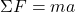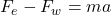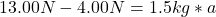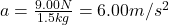## What is the acceleration of a 1.5 kg football thrown with a force of 13.00 N to the east with a westward wind opposing it with a

Question

What is the acceleration of a 1.5 kg football thrown with a force of 13.00 N to the

east with a westward wind opposing it with a force of 4.000 N?

in progress 0
2 months 2021-07-21T11:49:25+00:00 1 Answers 0 views 0

The acceleration of football is 6.00 m/s².

Explanation:

The acceleration can be calculated using the following equation:Where:

F: is the force

m: is the mass

a: is the accelerationTherefore, the acceleration of football is 6.00 m/s².

I hope it helps you!